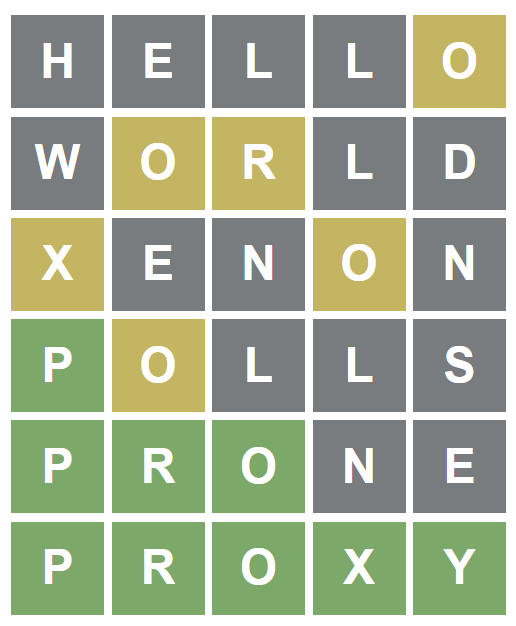# Optimal coverage for Wordle with Monte Carlo methods - Part I

Marton Trencseni - Wed 19 January 2022 - Machine Learning

## Introduction

Wordle is a simple 5x5 word guessing game. It's free, a new puzzle is posted every day. You have to guess an unknown 5 letter word, like PROXY. You have 5 guesses, and after each guess, the game tells you if you got one of the letters, but it's not at the right position (brown), or it's the right letter in the right position (green). Only words from the Wordle dictionary are accepted as guesses, ie. HELLO and WORLD are valid guesses, but ABCDE is not an accepted guess.The list of Wordle dictionary words is known, it's a set of 12,972 words.

## Optimal coverage challenge

One strategy is to analyze the set of words, frequencies of letters, and given what we know so far, pick the word [from the Wordle dictionary] to guess that maximizes the average information gain of the next guess, assuming that the target word is randomly selected [from the Wordle dictionary]. One of the inputs to this is an assumption how the player values knowing the correct position of the letters (green) versus just knowing the set of letters making up the word (brown).

There is a coincidence (?) in the structure in the game: the english alphabet is 26 words, and we can guess a total of 25 characters. If we can find 5 words which share no identical characters, then we can always use these 5 words as our 5 guesses, and assuming the target word has N unique letters, we will always no at least N-1. Eg. if the word is HELLO, and after our 5 guesses we know that H E L and O are in the words, then:

• either the word has a duplicate letter, one of those 4
• the word has a fifth unique letter, the 26th letter that was not included in our 5 guesses (which covered 25)

We don't know which of the above is the case, but most of the time we can assume a reasonable player will be able to guess the target word at this point, also taking into account that on average a few letters' position will be known.

So the question is: from the Wordle dictionary, can we find 5 words such that all 25 letters are unique? If not, what is the maximum achievable unique-count?

## Monte Carlo approach

The simplest Monte Carlo approach is to just randomly pick 5 words from the dictionary, and check how many unique letters we have:

words = ["cigar", "rebut", "sissy", ... ] # the Wordle dictionary
num_tests = 1000*1000
for _ in range(num_tests):
wordlist = random.sample(words, 5) # 5 random words
letters = set(list(''.join(wordlist)))
if len(letters) >= 21:
print(len(letters), wordlist)


It will print something like:

21 ['lotsa', 'finks', 'judge', 'macho', 'warby']
21 ['muzak', 'vales', 'letch', 'bilgy', 'fjord']
21 ['fagot', 'cushy', 'pawks', 'melon', 'diver']
21 ['miter', 'munch', 'pawky', 'bodge', 'lifes']
22 ['kight', 'flyby', 'roven', 'clasp', 'dwaum']


Let's try to be a little smarter.

## Parallelization

The simplest, still brute force improvement is to run the same thing, but in parallel. I have a 12-core (24 threads) CPU, so I can run 16x parallelism without impact the usability of my computer:

def solve_wordle(num_tests=1000, random_seed=0):
random.seed(random_seed * 131071)
solutions = []
for _ in range(num_tests):
wordlist = random.sample(words, 5) # 5 random words
letters = set(list(''.join(wordlist)))
if len(letters) >= 21:
solutions.append(wordlist)
return solutions

def flatten_list(li):
return [item for sublist in li for item in sublist]

def clean_solutions(solutions):
return [t.split(',') for t in sorted({','.join(sorted(c)) for c in solutions})]

n_jobs = 16
num_tests = 1*1000*1000
solutions = Parallel(n_jobs=n_jobs)(delayed(solve_wordle)
(num_tests=num_tests, random_seed=i)
for i in range(n_jobs))
solutions = clean_solutions(flatten_list(solutions))
for wordlist in solutions:
num_unique_letters = len(set(list(''.join(wordlist))))
print(f'{num_unique_letters}-unique-letter solution: {wordlist}')
if num_unique_letters == 25:
print('*** JACKPOT! ***')
print(f'Found {len(solutions)} solutions...')


The ipython notebook is on Github. Prints something like:

21-unique-letter solution: ['admix', 'itchy', 'ovels', 'tupik', 'wrung']
21-unique-letter solution: ['adzed', 'boxty', 'brugh', 'vinyl', 'wacks']
...
21-unique-letter solution: ['fyces', 'goban', 'lurve', 'mewed', 'piths']
21-unique-letter solution: ['light', 'packs', 'vends', 'wauff', 'womby']
Found 47 solutions...


## Conclusion

In the next part, I will show an improved Monte Carlo approach, which finds about one 24-unique-letter solution every second.